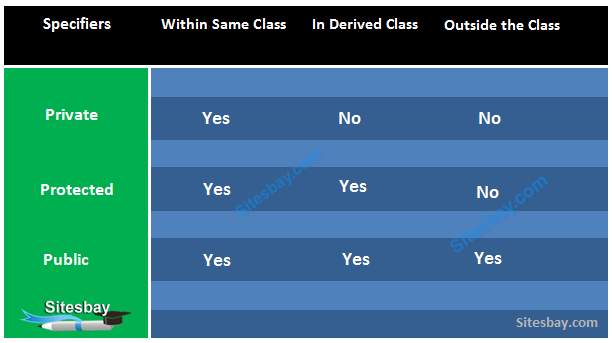# Access Specifiers in C++

## Access Specifiers in C++

Access specifiers in C++ define how the members of the class can be accessed. C++ has 3 new keywords introduced, namely.

• public
• private
• protectedThe keywords public, private, and protected are called access specifiers. A class can have multiple public, protected, or private labeled sections.

Note: By default, all members and function of a class is private i.e if no access specifier is specified.

## Syntax

```
class
{
private:
// private members and function
public:
// public members and function
protected:
// protected members and function
};
```

### Public Access Specifier in C++

Public class members are accessible out side the class and it is available for every one.

## Syntax

```
class Public_Access_Specifier
{
public:             // public access specifier
int a;             // Data Member Declaration
void display();     // Member Function declaration
}
```

### Private Access Specifier in C++

Private class members are accessible with the class and it is not accessible out side the class. If some one try to access out side the it gives compile time error. By default class variables and member functions are private.

## Syntax

```
class Private_Access_Specifier
{
private:           // private access specifier
int a;            // Data Member Declaration
void display();    // Member Function declaration
}
```

## Example

```
#include<iostream.h>
#include<conio.h>

class A
{
private:
int a;
public:
int b;

public:

void show()
{
a=10 ;
b=20;
clrscr();
//Every members can be access here, same class
cout<<"\nAccessing variable within the class"<<endl;

cout<<"Value of a: "<<a<<endl;
cout<<"Value of b: "<<b<<endl;
}
};

void main()
{
A obj; // create object
obj.show();

cout<<"\nAccessing variable outside the class"<<endl;
//'a' cannot be accessed as it is private
//cout<<"value of a: "<<obj.a<<endl;

//'b' is public as can be accessed from any where
cout<<"value of b: "<<obj.b<<endl;

getch();
}
```

Note: If here, we access variable a in side main method it will give compile time error

## Output

```
Accessing variable within the class
value of a: 10
value of b: 20
value of c: 30

Accessing variable outside the class
Value of b: 20
```

### Protected Access Specifier in C++

It is similar to private access specifier. It makes class member inaccessible outside the class. But they can be accessed by any subclass of that class.

## Syntax

```
class Protected_Access_Specifier
{
protected:         // protected access specifier
int a;            // Data Member Declaration
void display();    // Member Function Declaration
}
```

### Access Specifier Example in C++

In below example I will show you all these access specifier public, private and protected.

## Access Specifier Example in C++

```
#include<iostream.h>
#include<conio.h>

//using namespace std;

class Declaration
{
private:
int a;
public:
int b;
protected:
int c;
public:

void show()
{
a=10;
b=20;
c=30;

//Every members can be access here, same class
cout<<"\nAccessing variable within the class"<<endl;

cout<<"Value of a: "<<a<<endl;
cout<<"Value of b: "<<b<<endl;
cout<<"Value of c: "<<c<<endl;
}
};

class Sub_class:public Declaration
{
public:
void show()
{
b=5;
c=6;
cout<<"\nAccessing variable in sub the class"<<endl;

// a is not accessible here it is private
//cout<<"Value of a: "<<a<<endl;
//b is public so it is accessible any where
cout<<"Value of b: "<<b<<endl;
//'c' is declared as protected, so it is accessible in sub class
cout<<"Value of c: "<<c<<endl;
}
};

void main()
{
clrscr();
Declaration d; // create object
d.show();

Sub_class s; // create object
s.show();    // Sub class show() function

cout<<"\nAccessing variable outside the class"<<endl;
//'a' cannot be accessed as it is private
//cout<<"value of a: "<<d.a<<endl;

//'b' is public as can be accessed from any where
cout<<"value of b: "<<d.b<<endl;

//'c' is protected and cannot be accesed here
//cout<<"value of c: "<<d.c<<endl;
getch();
}
```

## Output

```
Accessing variable within the class
value of a: 10
value of b: 20
value of c: 30

Accessing variable in sub class
value of b: 5
value of c: 6

Accessing variable outside the class
Value of b: 20
```